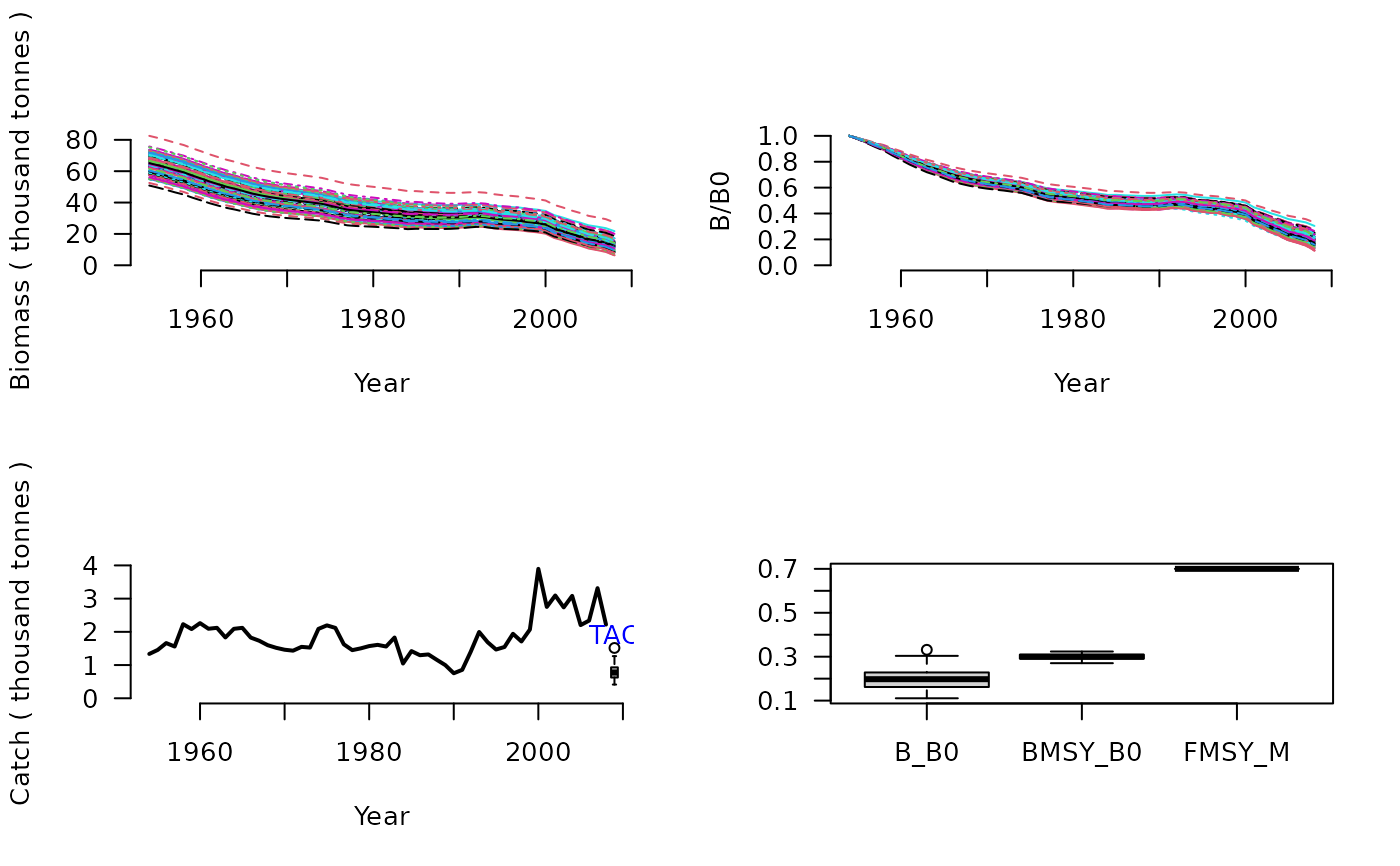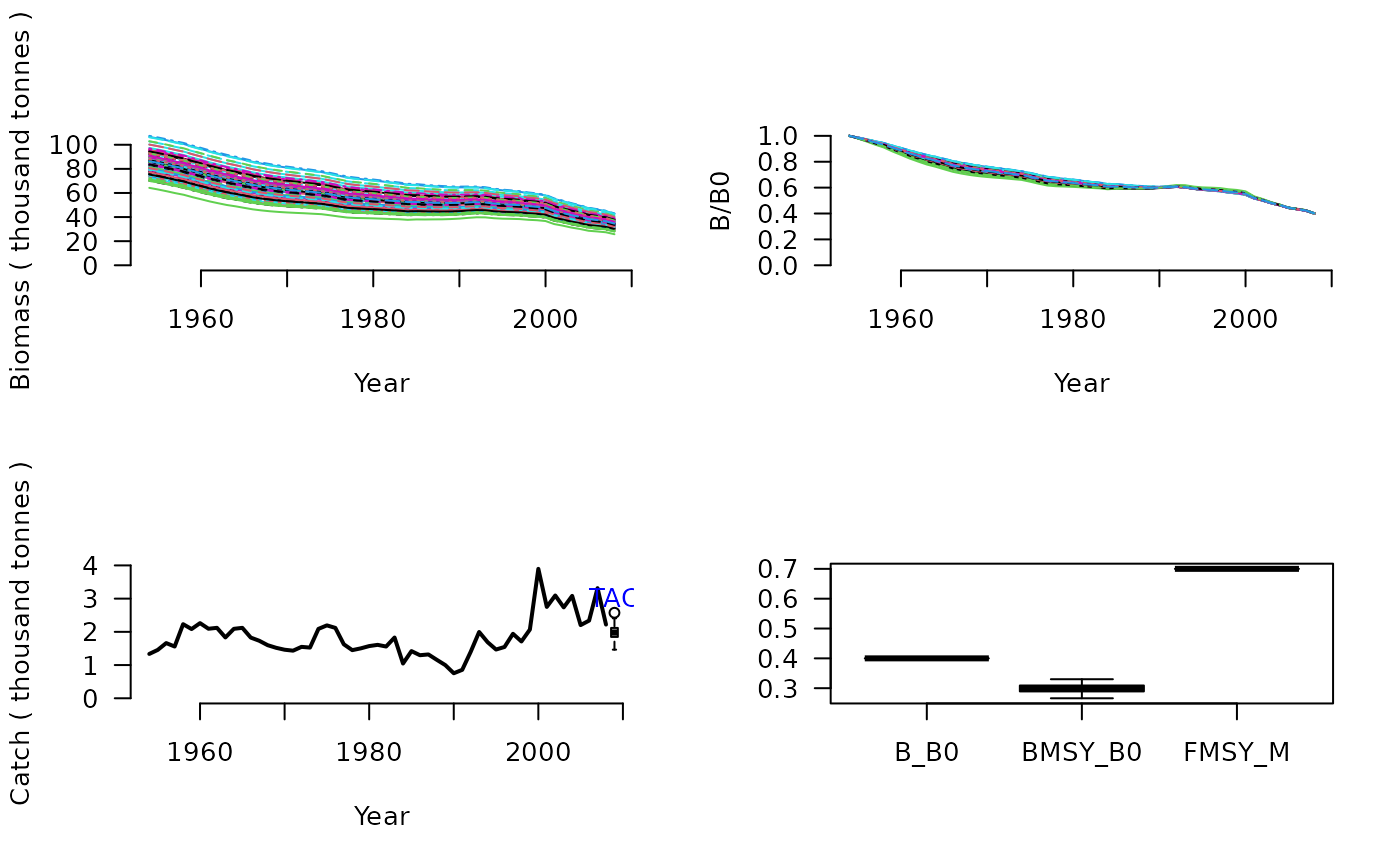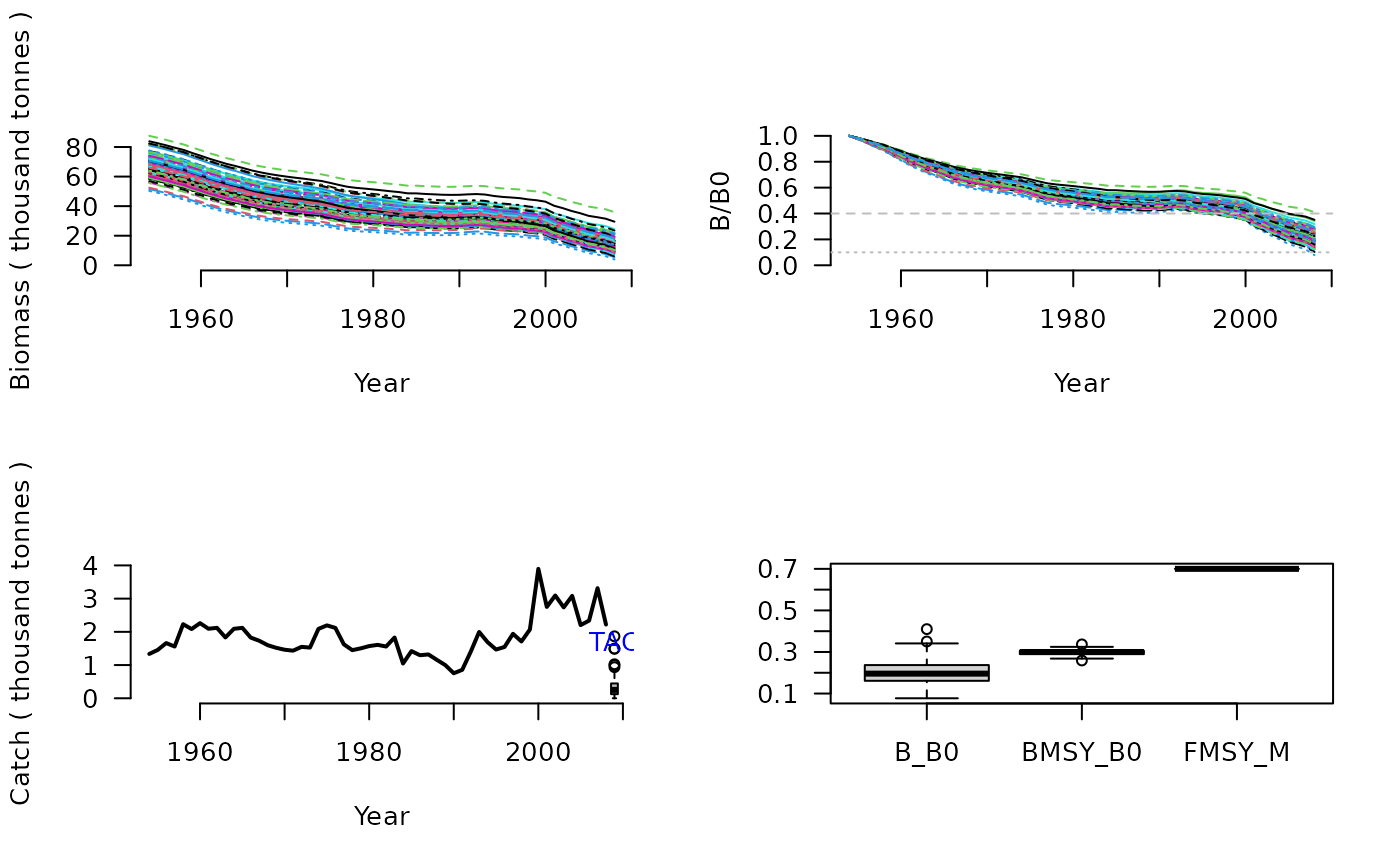Depletion-Based Stock Reduction Analysis (DB-SRA) is a method designed for determining a catch limit and management reference points for data-limited fisheries where catches are known from the beginning of exploitation. User prescribed BMSY/B0, M, FMSY/M are used to find B0 and therefore the a catch limit by back-constructing the stock to match a user specified level of stock depletion.

DBSRA(x, Data, reps = 100, plot = FALSE)

DBSRA_40(x, Data, reps = 100, plot = FALSE)

DBSRA4010(x, Data, reps = 100, plot = FALSE)

## Arguments

x

A position in the data object

Data

A data object

reps

The number of stochastic samples of the MP recommendation(s)

plot

Logical. Show the plot?

## Value

An object of class Rec-class with the TAC slot populated with a numeric vector of length reps

## Details

DB-SRA assumes that a complete time-series of catch from the beginning of exploitation is available. Users prescribe estimates of current depletion $$(D)$$, biomass at MSY relative to unfished $$\left(\frac{B_\textrm{MSY}}{B_0}\right)$$, the natural mortality rate $$(M)$$, and the ratio fishing mortality at MSY to M $$\left(\frac{F_{\textrm{MSY}}}{M}\right)$$.

You may have noticed that you -the user- specify three of the factors that make the quota recommendation. So this can be quite a subjective method. In the MSE the MSY reference points (e.g., $$\left(\frac{F_\textrm{MSY}}{M}\right)$$) are taken as the true value calculate in the MSE with added uncertainty specified in the Obs object (e.g Obs@FMSY_Mbiascv).

The catch limit, for the Base Version, is calculated as: $$\textrm{TAC} = M \frac{F_{\textrm{MSY}}}{M} D B_0$$

## Functions

• DBSRA: Base Version. TAC is calculated assumed MSY harvest rate multiplied by the estimated current abundance (estimated B0 x Depletion)

• DBSRA_40: Same as the Base Version but assumes 40 percent current depletion (Bcurrent/B0 = 0.4), which is more or less the most optimistic state for a stock (ie very close to BMSY/B0 for many stocks).

• DBSRA4010: Base version paired with the 40-10 rule that linearly throttles back the TAC when depletion is below 0.4 down to zero at 10 percent of unfished biomass.

## Note

The DB-SRA method of this package isn't exactly the same as the original method of Dick and MacCall (2011) because it has to work for simulated depletions above BMSY/B0 and even on occasion over B0. It also doesn't have the modification for flatfish life histories that has previously been applied by Dick and MacCall (2011).

## Required Data

See Data-class for information on the Data object

DBSRA: BMSY_B0, Cat, Dep, FMSY_M, L50, vbK, vbLinf, vbt0

DBSRA_40: BMSY_B0, Cat, FMSY_M, L50, vbK, vbLinf, vbt0

DBSRA4010: BMSY_B0, Cat, Dep, FMSY_M, L50, vbK, vbLinf, vbt0

## Rendered Equations

See Online Documentation for correctly rendered equations

T. Carruthers

## Examples

DBSRA(1, MSEtool::ourReefFish, plot=TRUE)#> TAC (median)
#>    0.7464144

DBSRA_40(1, MSEtool::ourReefFish, plot=TRUE)#> TAC (median)
#>     2.035598
DBSRA4010(1, MSEtool::ourReefFish, plot=TRUE)#> TAC (median)
#>     0.258382